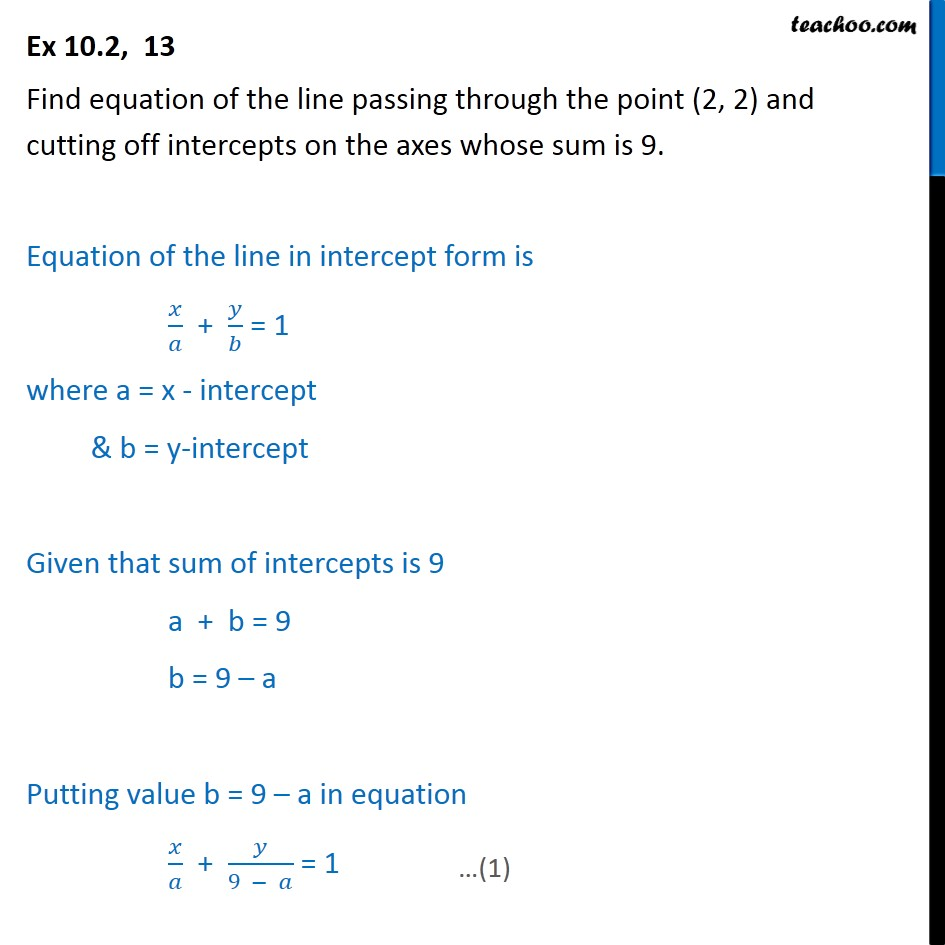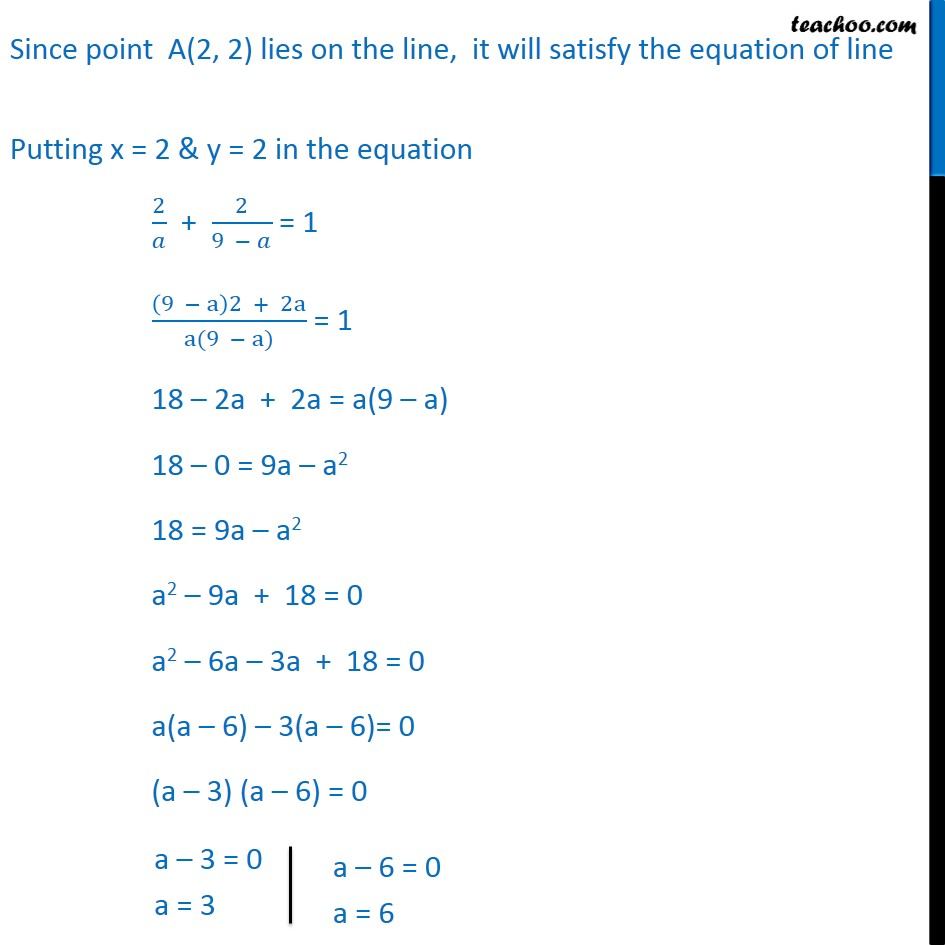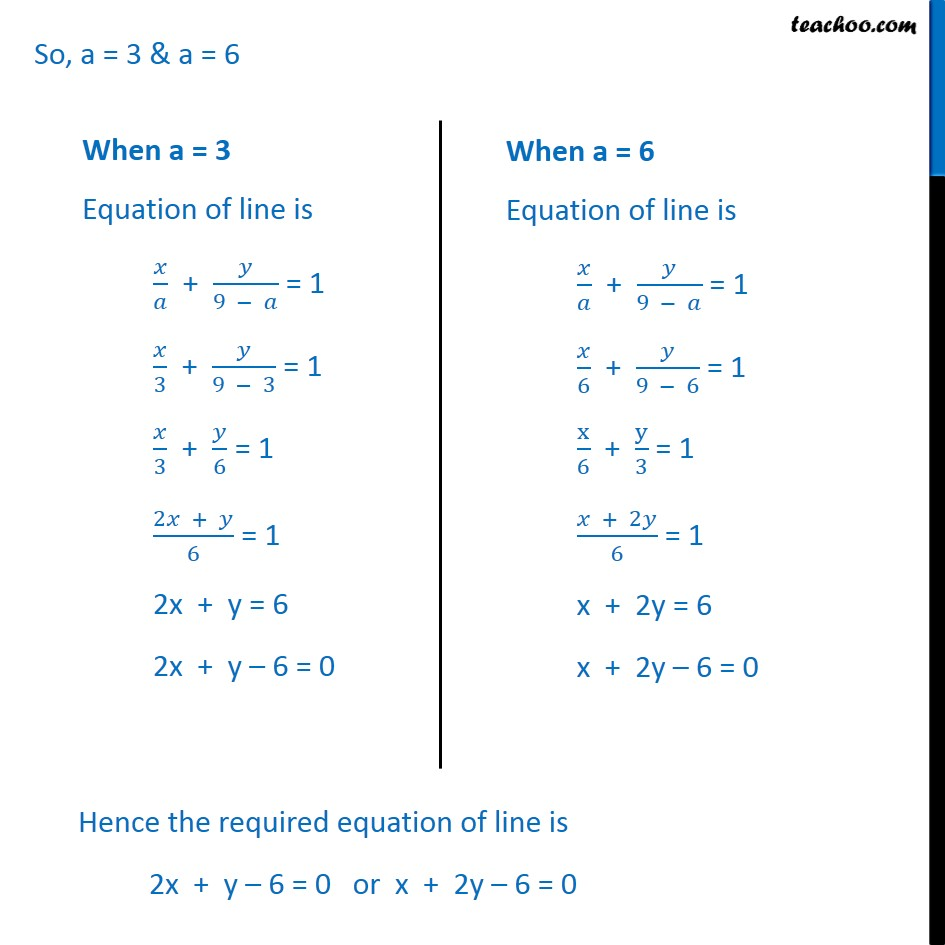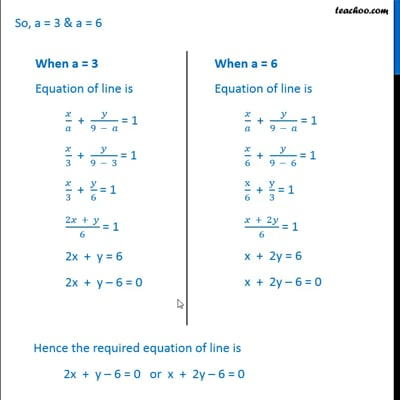Ex 10.2

Chapter 10 Class 11 Straight Lines
Serial order wiseThis video is only available for Teachoo black users

Solve all your doubts with Teachoo Black (new monthly pack available now!)

### Transcript

Ex 10.2, 13 Find equation of the line passing through the point (2, 2) and cutting off intercepts on the axes whose sum is 9. Equation of the line in intercept form is / + / = 1 where a = x - intercept & b = y-intercept Given that sum of intercepts is 9 a + b = 9 b = 9 a Putting value b = 9 a in equation / + /(9 ) = 1 Since point A(2, 2) lies on the line, it will satisfy the equation of line Putting x = 2 & y = 2 in the equation 2/ + 2/(9 ) = 1 ((9 a)2 + 2a)/(a(9 a)) = 1 18 2a + 2a = a(9 a) 18 0 = 9a a2 18 = 9a a2 a2 9a + 18 = 0 a2 6a 3a + 18 = 0 a(a 6) 3(a 6)= 0 (a 3) (a 6) = 0 So, a = 3 & a = 6